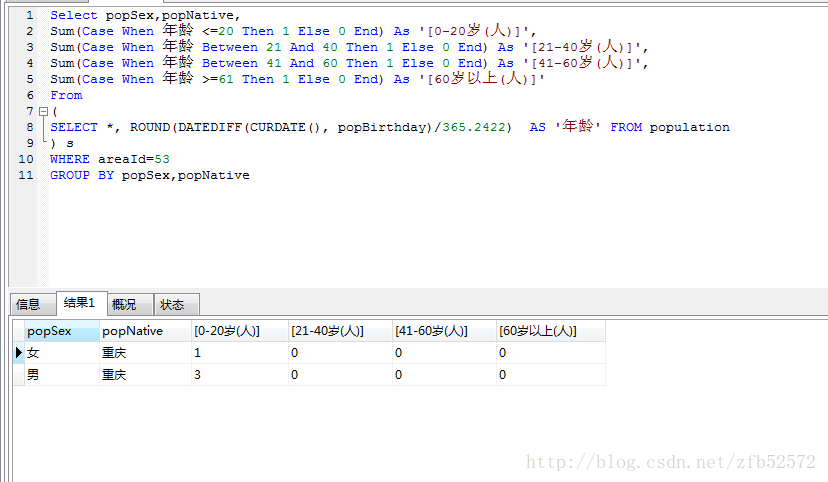# SQL语句查询年龄分段分组查询

1.SELECT *, ROUND(DATEDIFF(CURDATE(), popBirthday)/365.2422)  AS '年龄' FROM population   ：这是获取当前日期，

2. Select popSex,popNative,
Sum(Case When 年龄 <=20 Then 1 Else 0 End) As '[0-20岁(人)]',
Sum(Case When 年龄 Between 21 And 40 Then 1 Else 0 End) As '[21-40岁(人)]',
Sum(Case When 年龄 Between 41 And 60 Then 1 Else 0 End) As '[41-60岁(人)]',
Sum(Case When 年龄 >=61 Then 1 Else 0 End) As '[60岁以上(人)]'
From
(
SELECT *, ROUND(DATEDIFF(CURDATE(), popBirthday)/365.2422)  AS '年龄' FROM population
) s
WHERE areaId=53
GROUP BY popSex,popNative---------------------

posted @ 2018-11-01 15:08  Nullnullisnull  阅读(2347)  评论(0编辑  收藏